Name:    TriangleQuiz

True/False
Indicate whether the statement is true or false.

1.

The medians and altitudes of an isosceles triangle are all concurrent.

Multiple Choice
Identify the choice that best completes the statement or answers the question.

2.

The diagonals of the square with vertices at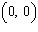,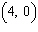,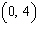, and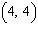meet at a point P. What are the coordinates of P?
 a.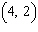c.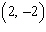b.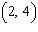d.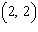3.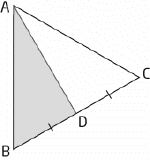The area of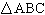is 22 square units. What is the area of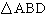?
 a. 5 square units c. 15 square units b. 11 square units d. 22 square units

4.

What is the perimeter of the triangle whose vertices are at the points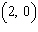,, and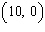?
 a. 11 units c. 18 units b. 15 units d. 21 units

5.

What is the area of the triangle whose vertices are at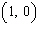,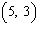, and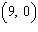?
 a. 6 square units c. 18 square units b. 12 square units d. 24 square units

6.

What is the equation of the right bisector of the line segment from A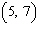to B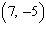?
 a.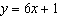c.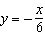b.d.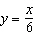7.

Which of the following points is the centroid of the triangle with vertices at A, B, and C?
 a.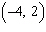c.b.d.